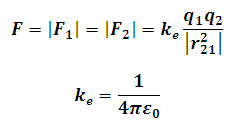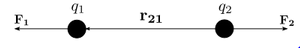# Coulomb's Law Calculator

This Calctown Calculator calculator calculates the electrostatic force of interaction between two point charges using coulomb's law.

Please enter the relative permittivity accordingly.

C
C
m

#### Result

NClick here to view image

where

F1, F2 are electrostatic forces acting on the respective point charges

r21 = distance between the point charges

q1, q2 charges on respective point charges

ke = coulomb's constant